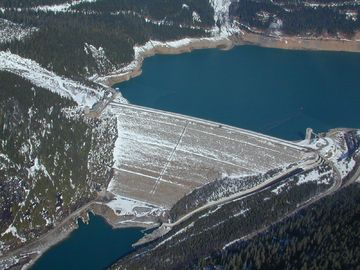# Energy from waterFigure 1. The Mica Dam in British Columbia is one example of how the energy in water can be harnessed for human use.

The energy from water can be harnessed to be useful in a variety of different ways through water wheels or in hydroelectricity generating facilities. As water moves through some body, such as a river, its potential and kinetic energy vary. Additionally, if the area through which the water is moving changes size the pressure can also change. A device such as a turbine, can harness the kinetic and potential energy to be transformed into a type of useable energy, such as electricity.

## Kinetic Energy of Water

The kinetic energy of water is a result of the speed or flow rate of the water. The relationship for the kinetic energy per unit volume of water is thus proportional to its velocity and can be expressed as:

where:

As well, from this expression for kinetic energy the power that can be harnessed from this kinetic energy can be expressed as:

where:

• is the power in watts per cubic meter (W)
• is the density of the fluid in kilograms per cubic meter (kg/m3)
• is the cross-sectional area of the flow in square meters (m2)
• is the velocity of the fluid in meters per second (m/s)

The result of the cubic relationship is that a flow with twice the velocity of a reference will have eight times the kinetic energy. This fact has a large influence on the placement of potential hydroelectric generators, as the best place to put them is where water is flowing most quickly.

## Potential Energy of Water

The potential energy of water is the energy the water obtains as a result of being at some elevation. Put simply, the head difference of water is what results in potential energy. The relationship for the potential energy per unit volume of water is thus proportional to its height and can be expressed as:

where:

• is the potential energy of the fluid in joules (J)
• is the volume of the fluid (m3)
• is the density of the fluid in kilograms per cubic meter (kg/m3)
• is the acceleration due to gravity in meters per second squared (m/s2)
• is the height of the fluid in meters (m)

As well, from this expression for potential energy the power that can be harnessed from this potential energy can be expressed as:

where:

• is the power in watts (W)
• is the density of fluid in kilograms per cubic meter (kg/m3)
• is the flow rate of the fluid in cubic meters per second (m3/s)
• is the acceleration due to gravity in meters per second squared (m/s2)
• is height difference between two points of the fluid flow in meters (m)

This expression is known as the hydroelectric power equation. The height difference between inlet and outlet in conventional generation is generally created by damming a river to create a reservoir. Water from the reservoir is then directed through turbines which convert the energy in the fluid to electrical energy. The amount of energy that can be converted is related to the efficiency of the turbine and electrical generator.

## Pressure

Pressure potential energy is the final type of energy that fluids exhibit. This term along with the two mentioned above can be combined in Bernoulli's equation to fully represent the energy of a flowing fluid. Pressure, P, is simply a measure of energy per unit volume, or energy density. This concept can be clarified in terms similar to that of potential energy using the relationship:

where:

• is the pressure of the fluid
• is the volume of the fluid

This expression can be thought of as a potential type energy as it represents the energy stored in a vessel of a given volume, containing a given amount of fluid stored at a certain pressure. This fluid has not been released, but when released this pressurized fluid has the ability to do work.

## Authors and Editors

Bethel Afework, Jordan Hanania, Kailyn Stenhouse, Jason Donev
Last updated: May 18, 2018
Get Citation